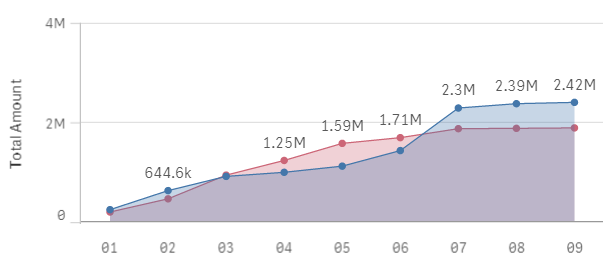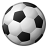# App Development

Announcements
Qlik Insider: SaaS capabilities for Data Integration and Analytics, May 25th! Register Now
cancel
Showing results for
Did you mean:Contributor II

## Ignore current selections within an aggr function

In Qlik Sense I'm using the following function as a measure to get a cumulative chart with two dimensions.

=aggr(rangesum(above(sum(TotalAmount), 0, rowno() )), Time.Year, [Time.Year Week])

That is working well and produces the graph shown below (I'm using some simple IF statements in the dimensions to constrain them to current/previous years and weeks to date).

Now I want to use set analysis to ignore current selections in the values. I can't figure out how to modify the function above to achieve that. Any suggestions?1 Solution

Accepted SolutionsMVP

=sum({1}aggr(rangesum(above(sum({1}TotalAmount), 0, rowno() )), Time.Year, [Time.Year Week]))

talk is cheap, supply exceeds demand
3 RepliesMVP

=sum({1}aggr(rangesum(above(sum({1}TotalAmount), 0, rowno() )), Time.Year, [Time.Year Week]))

talk is cheap, supply exceeds demandContributor II
Author

Thanks Gysbert,

If I'm honest, I don't know exactly why the solution involved putting a sum() around all of it... but it does feel right and it does work.

I had tried putting {1} before TotalAmount. My grasp of set analysis, aggr and rangesum is still a bit tenuous.

Best,

MattCreator

Adding a prefix of Sum({1} to the aggr function is still a solution in 2020.

Thanks